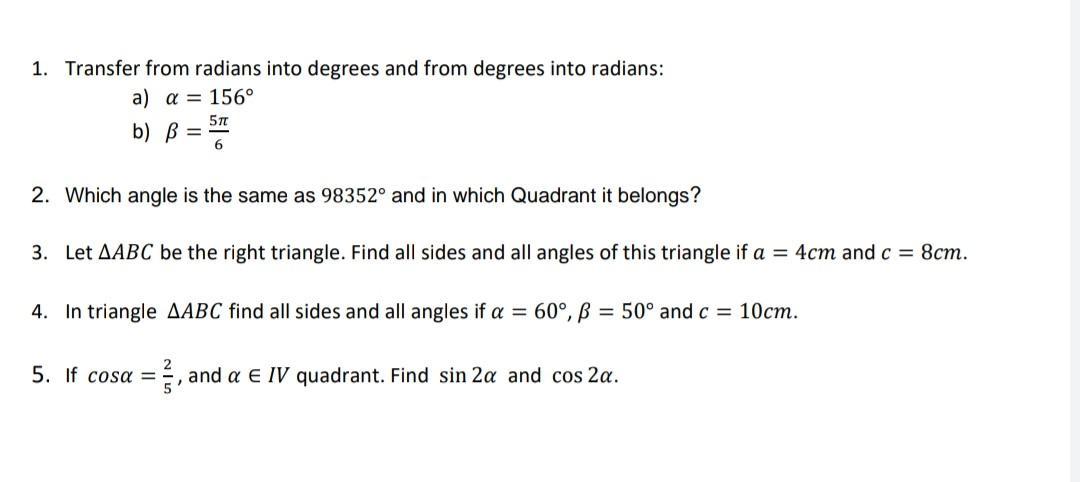# (Solved): 1. Transfer from radians into degrees and from degrees into radians: a) =156 b) =65 ...1. Transfer from radians into degrees and from degrees into radians: a) b) 2. Which angle is the same as and in which Quadrant it belongs? 3. Let be the right triangle. Find all sides and all angles of this triangle if and . 4. In triangle find all sides and all angles if and . 5. If , and quadrant. Find and .

We have an Answer from Expert

radian measure = (degree measure × ?)/180
Steps
Step 1: Plug the angle value, in degrees, in the formula above:
radian measure = (156 × ?)/180
Step 2: Rearrange the terms:
radian measure = ? × 156/180
Step 3: Reduce or simplify the fraction of ? if necessary
Calculating the gcd of 156 and 180 [gcd(156,180)], we've found that it equals 12. So, we can simplify this fraction by reducing it to lowest terms:
Dividing both numerator and denominator by the gcd 12, we have:
? × 156÷12/180÷12 which equals
13?/15 radian, after reducing the fraction to lowest terms.
Note: 13?/15 rad is the same as:
0.86666666666667? radian (as a decimal in terms of ?)
2.7227136331112 radian (as a real number)

-5?/6 radians is equivalent to -150°. Since ? radians is equal to 180°, we can plug 180° in for ? radians in -5?/6 radians, and then simplify to convert to degrees. We get that -5?/6 radians is equivalent to -150°.
We have an Answer from Expert